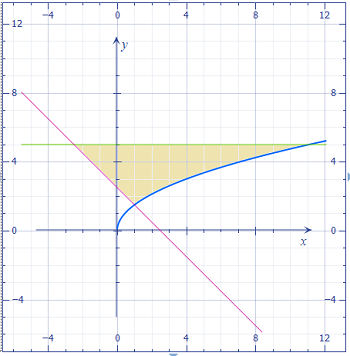# Sketch the region enclosed by the given curves. Decide whether it is easier to integrate with...

## Question:

Sketch the region enclosed by the given curves. Decide whether it is easier to integrate with respect to x or y. Then find the area of the region.

{eq}2y=3\sqrt x, \ y=5 {/eq} and {eq}2y+2x=5 {/eq}

## Double Integrals:

Recall that in general we can use a double integral to find the area of a region. Recall also that there are two different ways to set up any double integral: one for each order of integration. To help determine which order will be most convenient to evaluate, we can always make a quick plot of the region.

The region is plotted below.Note that we can bound {eq}y {/eq} over a particular region and use the curves to bound {eq}x {/eq}, so that's what we want to do. We can write

{eq}\begin{align*} 2y &= 3 \sqrt x \\ \sqrt x &= \frac23 y \\ x &= \frac49 y^2 \end{align*} {/eq}

and

{eq}\begin{align*} 2y + 2x &= 5 \\ y + x &= \frac52 \\ x &= \frac52 - y \end{align*} {/eq}

So {eq}\frac52 - y \leq x \leq \frac49 y^2 {/eq}. To find the lower bound on {eq}y {/eq} explicitly we need to know where the two curves intersect.

{eq}\begin{align*} \frac52 - y &= \frac49 y^2 \\ \frac{45}{2} - 9y &= 4y^2 \\ 4y^2 - 9y + \frac{45}{2} &= 0 \\ \left( 2y-3 \right) \left( 4y+15 \right)&= 0 \\ y &= - \frac{15}{4},\ \frac32 \end{align*} {/eq}

We throw away the negative value since we are in the first quadrant, so {eq}y \in [ \frac32, 5 ] {/eq}. Then the area between the curves is

{eq}\begin{align*} A &= \int_{3/2}^5 \int_{5/2 - y}^{4y^2/9} dx\ dy \\ &= \int_{3/2}^5 \frac49 y^2 - \frac52 + y\ dy \\ &= \left [ \frac{4}{27} y^3 - \frac52y + \frac12y^2 \right ]_{3/2}^5 \\ &= \frac{4}{27} \left( 5^3 - \left( \frac32 \right)^3 \right) - \frac52 \left( 5 - \frac32 \right) + \frac12 \left( 5^2 - \left( \frac32 \right)^2 \right) \\ &= \frac{4459}{216} \\ &\approx 20.6435 \end{align*} {/eq}